# Solution June 28, 2007

### Problem 1

Find all positive integers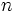$n\,$ such that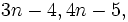$3n-4,4n-5,\,$ and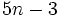$5n-3\,$ are all prime numbers.

### Solution 1

Remember that 2 is the only even prime. We consider two cases.

• n even: Then 3n − 4 is an even prime so that 3n − 4 = 2 and n = 2. The resulting numbers 2,3,7 are indeed prime so this is a solution.
• n odd: Then 5n − 3 is an even prime, and 5n − 3 = 2. This implies n = 1 which isn't a solution because 3 − 4 = − 1 is not a prime number.

So n = 2 is the only solution to the problem.

### Problem 2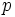$p\,$ and$q\,$ are prime numbers.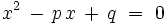$x^2\, -\,p\,x\, +\,q\ =\ 0$ has distinct rational roots. Find all$p\,$ and$q\,$ which work.

### Solution 2

We can write x2px + q = (xa)(xb) where a and b are the roots of the polynomial.

By the rational roots theorem (http://en.wikipedia.org/wiki/Rational_root_theorem) (if this is too scary, see the note below), the roots of x2px + q = 0 are integers that divide q.

The product of the two roots must be q so we have a = − 1,b = − q or a = 1,b = q.

The sum of the two roots is equal to p which rules out the first possibility, because p > 0.

Now we have p = q + 1 where p and q are both prime. The only possibility for that is p = 3 and q = 2. That answers the problem.

Note: Instead of using the rational roots theorem, we can have a closer look at the quadratic formula. The roots of x2px + q are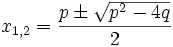$x_{1,2} = \frac{p\pm\sqrt{p^2-4q}}2$

They are only rational when p2 − 4q is a perfect square. If p is even then p2 − 4q is even and so is its square root. Similarily, if p is odd then p2 − 4q is odd and its square root is odd. In either case,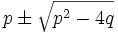$p\pm\sqrt{p^2-4q}$ are even, so the roots are integers if they are rational.